Refer to our Texas Go Math Grade 7 Answer Key Pdf to score good marks in the exams. Test yourself by practicing the problems from Texas Go Math Grade 7 Module 8 Quiz Answer Key.

8.1 Writing Two-Step Equations

Question 1.
Jerry started doing sit-ups every day. The first day he did 15 sit-ups. Every day after that he did 2 more sit-ups than he had done the previous day. Today Jerry did 33 sit-ups. Write an equation that could be solved to find the number of days Jerry has been doing sit-ups since the first day.
We have to sum the number of sit-ups Jerry did first day and x times 2,
where x represents the number of days he has been doing sit-ups since the first day, and that sum shouLd be number of sit-ups Jerry did today.
15 + 2x = 33 Subtract 15 from both sides.
2x = 33 – 15
2x = 18 Divide each side by Z
$$\frac{2 x}{2}$$ = $$\frac{18}{2}$$
x = 9
Jerry has been doing sit-ups for 9 days and plus one first day when he started.
Hence, he has been doing sit-ups for 10 days successive.

8.2 Solving Two-Step Equations

Solve.

Question 2.
5n + 8 – 43 ____________
Subtract 8 from both sides.
5n + 8 – 8 = 43 – 8
5n = 35 Divide both sides by 5.Question 3.
$$\frac{y}{6}$$ – 7 = 4
$$\frac{y}{6}$$ – 7 + 7 = 4 + 7
$$\frac{y}{6}$$ = 11 Multiply both sides by 6.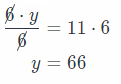Question 4.
8w – 15 = 57 __________
8w – 15 + 15 = 57 + 15
8m = 72 Divide both sides by 8.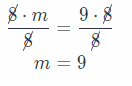Question 5.
$$\frac{g}{3}$$ + 11 = 25 ___________
Subtract 11 from both sides
$$\frac{g}{3}$$ + 11 – 11 = 25 – 11
$$\frac{g}{3}$$ = 14 Multiply both sides by 3.Question 6.
$$\frac{f}{5}$$ – 22 = -25 _________
$$\frac{f}{5}$$ – 22 + 22 = -25 + 22
$$\frac{f}{5}$$ = -3 Multiply both sides by 5.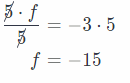Question 7.
-4p + 19 = 11 _________
Subtract 19 from both sides
-4p + 19 – 19 = 11 – 19
-4p = -8 Divide both sides by (-4)8.3 Writing Two-Step Inequalities

Question 8.
Eddie scored at least 27 points more than half of what Duncan scored. Eddie scored 58 points. Write an inequality that could be solved to find the numbers of points that Duncan could have scored.
Let the Duncan scored be x and Eddie scored be y
It is given in problem that Eddie scored at least 27 points more than half of what Duncan scored. So the inequality will be:
y ≥ $$\frac{x}{2}$$ + 27
Now it is also given that Eddie has scored 58 points, so inequality wiLL be:
$$\frac{x}{2}$$ + 27 ≤ 58

8.4 Solving Two-Step inequalities

Solve.

Question 9.
2s + 3 > 15 ________
Subtract 3 from both sides.
2s + 3 – 3 > 15 – 3 Divide both sides by 2.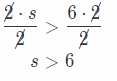Question 10.
$$\frac{d}{12}$$ – 6 < 1 ________
$$\frac{d}{12}$$ – 6 + 6 < 1 + 6
$$\frac{d}{12}$$ < 7 Multiply both sides by 12.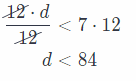Question 11.
6w – 18 ≥ 36 ________
6w – 18 + 18 ≥ 36 + 18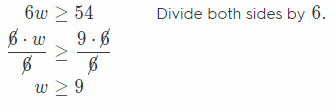Question 12.
$$\frac{z}{4}$$ + 22 ≤ 38
Subtract 22 from both sides
$$\frac{z}{4}$$ + 22 – 22 ≤ 38 – 22
$$\frac{z}{4}$$ ≤ 16 Multiply both sides by 4.Question 13.
$$\frac{b}{9}$$ – 34 < -36 ___
$$\frac{b}{9}$$ – 34 + 34 < -36 + 34
$$\frac{b}{9}$$ < -2 Multiply both sides by 9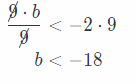Question 14.
-2p + 12 > 8 ________
Divide both sides by (-2), and reverse the direction of inequality, due to the division with a negative number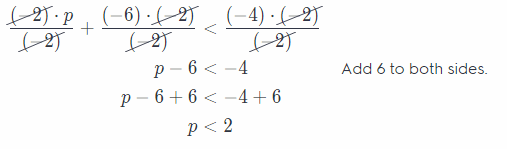Essential Question

Question 15.
How can you use two-step equations and inequalities to represent and solve real-world problems?
Using two-step equations and inequalities let you solve real-world problems for multiple pieces which have the
same values. For example, you have 8 dozens of doughnuts and you wanted to determine the cost of each dozen.
Dividing the total amount of the doughnuts by 8 would give you the cost of each dozen. Also when you wanted to
know the maximum numbers you could buy when you only have a limited amount to use.

Using two-step equations and inequalities let you determine the value of each for multiple pieces or determine the maximum numbers you could buy with a limited amount to use.

Texas Go Math Grade 7 Module 8 Mixed Review Texas Test Prep Answer Key

Texas Test Prep

Selected Response

Question 1.
A taxi cab costs $1.50 for the first mile and$0.75 for each additional mile. Which equation could be solved to find
how many miles you can travel in a taxi for $10, if x is the number of additional miles? (A) 1.5x + 0.75 = 10 (B) 0.75x + 1.5 = 10 (C) 1.5x – 0.75 = 10 (D) 0.75x – 1.5 = 10 Answer: (B) 0.75x + 1.5 = 10 Question 2. Tony operates a skate rental company. He charges an equipment fee of$3 plus $6 per hour. Which equation represents this linear relationship? (A) y = -6x + 3 (B) y = 3x + 6 (C) y = -6x + 3 (D) y = 3x – 3 Answer: (A) y = -6x + 3 Question 3. Which evaluation has x = 8 for a solution? (A) 2x + 3 = 13 (B) 4x + 6 = 38 (C) 3x – 5 = 29 (D) 5x – 8 = 48 Answer: (B) 4x + 6 = 38 Question 4. Which inequality has the following graphed solution?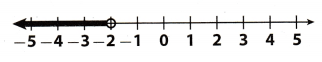(A) 3x – 8 ≤ 2 (B) 4x + 12 < 4 (C) 2x + 5 ≤ 1 (D) 3x + 6 < 3 Answer: (C) 2x + 5 ≤ 1 Question 5. Which represents the solution for the inequality 3x – 7 > 5? (A) x < 4 (B) x ≤ 4 (C) x > 4 (D) x ≥ 4 Answer: (C) x > 4 Question 6. The 30 members of a choir are trying to raise at least$1,500 to cover travel costs to a singing camp. They have already raised \$600. Which inequality could you solve to find the average amounts each’ member can raise in order to meet the goal?
(A) 30x + 600 ≥ 1,500
(B) 30x + 600 ≥ 1,500
(C) 30x + 600 < 1,500
(D) 30x + 600 ≤ 1,500
(B) 30x + 600 ≥ 1,500

Gridded Response

Question 7.
Mrs. Drennan keeps a bag of small prizes to distribute to her students. She likes to keep at least three times as many prizes in the bag as she has students. The bag currently has 72 prizes in it. Mrs. Drennan has 26 students. What is the least amount of prizes Mrs. Drennan needs to buy?